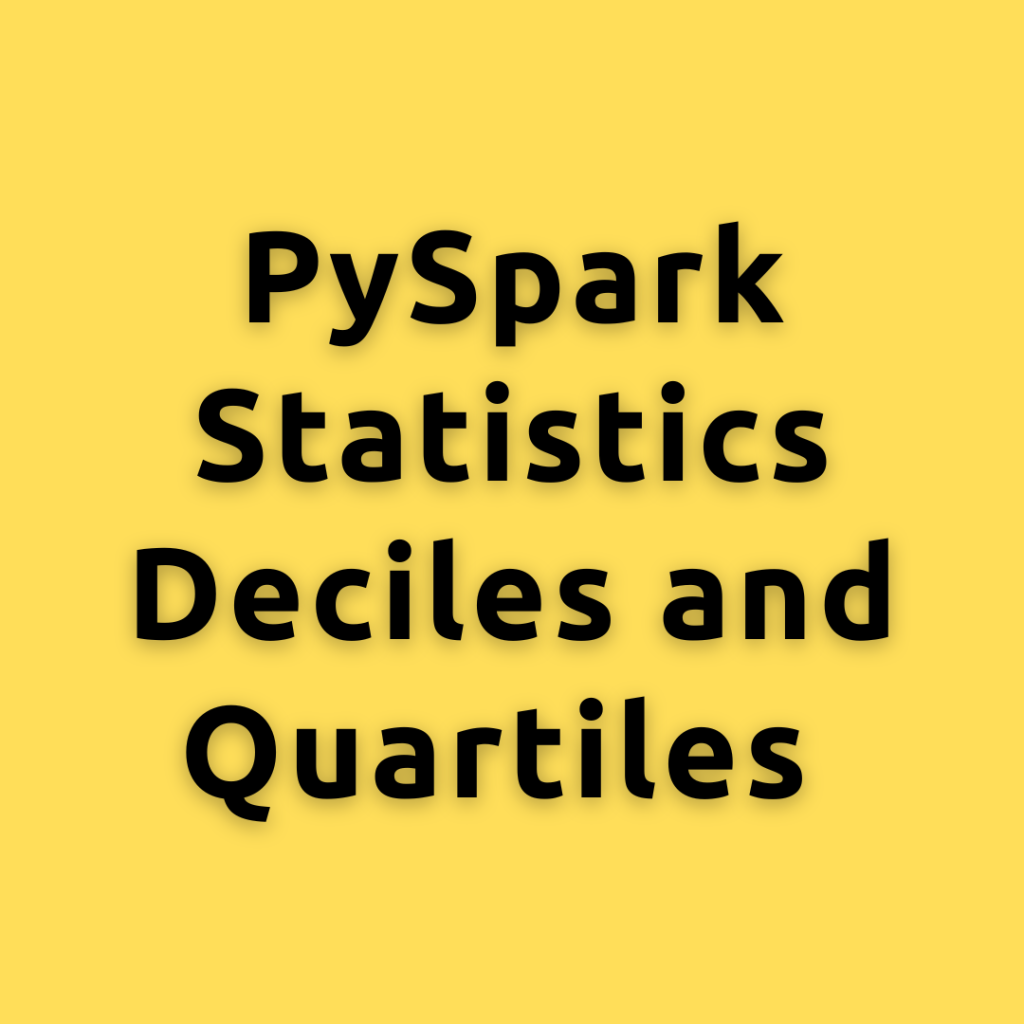## PySpark Statistics Deciles and Quartiles – Understanding Deciles and Quartiles a Deep Dive with PySpark

Let’s dive into the concept of deciles and quartiles and how to calculate them in PySpark. When analyzing data, it’s important to understand the distribution of the data. One way to do this is by calculating the deciles and quartiles. What are Deciles? Deciles divide a set of data into 10 equal parts. For example, …